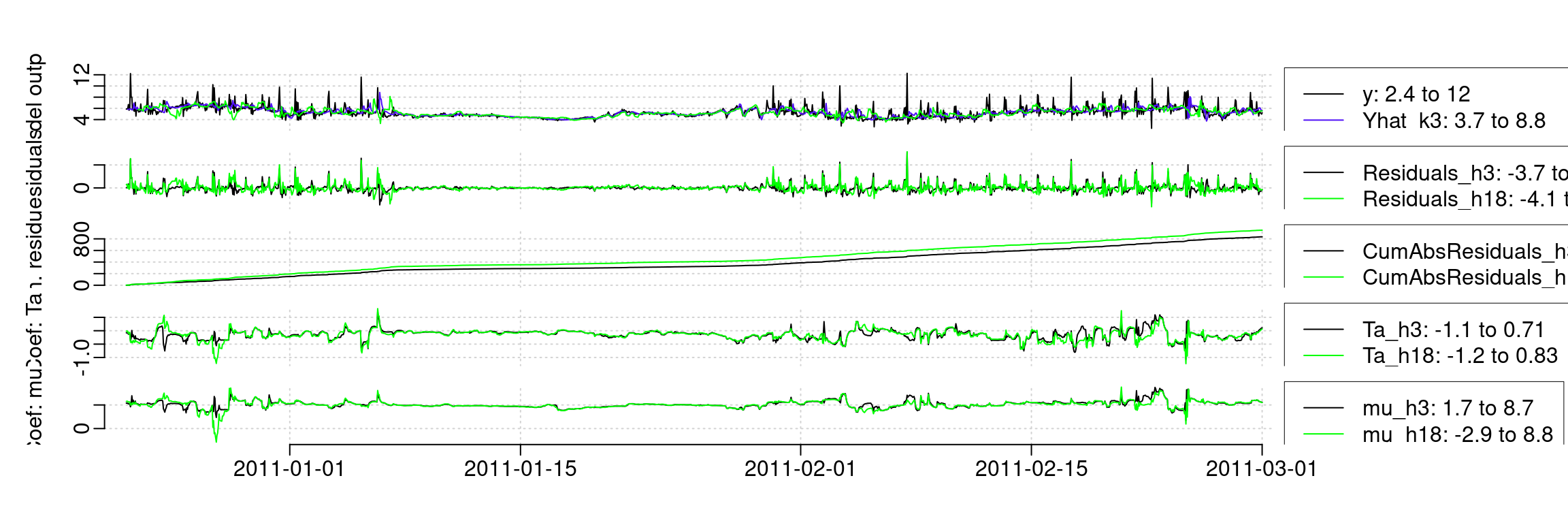In this vignette we provide a few tricks making it easier to work with the onlineforecast package.

## Caching optimization results

# Load the package and data
library(onlineforecast)
D <- subset(Dbuilding, c("2010-12-15", "2011-01-01"))
D$y <- D$heatload

# Define a simple model
model <- forecastmodel$new() model$add_inputs(Ta = "Ta", mu = "one()")
model$add_regprm("rls_prm(lambda=0.99)") # Period to include in the evaluation of the score function D$scoreperiod <- in_range("2010-12-20", D$t) # And the sequence of horizons to fit for model$kseq <- 1:6
# Add bounds for lambda (lower, init, upper)
model$add_prmbounds(lambda = c(0.9, 0.98, 0.999)) Optimize the parameter and see the result: # The optimization optimization of lambda rls_optim(model, D)$par
##     lambda
##      0.996

Instead of calculating the result each time, then cache the result:

tstart <- Sys.time()
# Caching can be done by providing a path
rls_optim(model, D, cachedir="cache")$par ## lambda ## 0.996 Sys.time() - tstart ## Time difference of 0.595 secs A file with the result of optim is now stored in the folder: # See the cache files dir("cache") ##  "rls_optim_f9e884084b84794d762a535f3facec85_543e21752d9c34bd22603fcfe88c0db6.RDS" Even in this very simple example it’s much faster to read the cache instead of doing the optimization: tstart <- Sys.time() # So running again the result is read from the file rls_optim(model, D, cachedir="cache")$par
##     lambda
##      0.996
Sys.time() - tstart
##     Time difference of 0.00391 secs

If anything affecting the results are changed, then optimization runs and the new result is cached:

# Change the lower bound for optimization
model$add_prmbounds(lambda = c(0.89, 0.98, 0.999)) # New optimization results are calculated and cached val <- rls_optim(model, D, cachedir="cache") dir("cache") ##  "rls_optim_f9e884084b84794d762a535f3facec85_543e21752d9c34bd22603fcfe88c0db6.RDS" ##  "rls_optim_f9e884084b84794d762a535f3facec85_807998b046c6188019321636d8302a6b.RDS" The cache files can easily be removed by: # To delete the cache folder unlink("cache", recursive=TRUE) This is also implemented in the lm_optim()’ function. ## Use plotly The plot_ts can be use plotly for plotting: D <- Dbuilding # Use plotly library(plotly) # usely=TRUE L <- plot_ts(D, c("heatload","Ta"), kseq=c(1,24), usely=TRUE, xlab="Time", ylabs=c("Heat (kW)","Temperature (C)")) ## Warning: arrange_() is deprecated as of dplyr 0.7.0. ## Please use arrange() instead. ## See vignette('programming') for more help ## This warning is displayed once every 8 hours. ## Call lifecycle::last_warnings() to see where this warning was generated. ## Warning: Can't display both discrete & non-discrete data on same axis ## Warning: Can't display both discrete & non-discrete data on same axis #' or exact same L <- plotly_ts(D, c("heatload","Ta"), kseq=c(1,24), xlab="Time", ylabs=c("Heat (kW)","Temperature (C)")) ## Warning: Can't display both discrete & non-discrete data on same axis ## Warning: Can't display both discrete & non-discrete data on same axis #' # From plotly the figures are returned and can be further manipulated # e.g. put the legend in the top by L[[length(L)]] <- L[[length(L)]] %>% layout(legend = list(x = 100, y = 0.98)) print(subplot(L, shareX=TRUE, nrows=length(L), titleY = TRUE)) ## Warning: Can't display both discrete & non-discrete data on same axis ## Warning: Can't display both discrete & non-discrete data on same axis #' \donttest{ #' D <- Dbuilding #' #' plotly_ts(D, c("heatload","Ta"), kseq=c(1,24)) #' plotly_ts(D, c("heatload","Ta$|Taobs$"), kseq=c(1,24)) #' } Also for a fit: D$scoreperiod <- in_range("2010-12-22", D$t) model <- forecastmodel$new()
model$output = "heatload" model$add_inputs(Ta = "Ta",
mu = "one()")
model$add_regprm("rls_prm(lambda=0.9)") model$kseq <- c(3,18)
fit1 <- rls_fit(NA, model, D, returnanalysis = TRUE)
##     ----------------
##     prm=NA, so current parameters are used.
#'
# Plot it
plot_ts(fit1)#'
# Plot it with plotly
plot_ts(fit1, usely=TRUE)
##     Warning: Can't display both discrete & non-discrete data on same axis

##     Warning: Can't display both discrete & non-discrete data on same axis

##     Warning: Can't display both discrete & non-discrete data on same axis

##     Warning: Can't display both discrete & non-discrete data on same axis

##     Warning: Can't display both discrete & non-discrete data on same axis

##     Warning: Can't display both discrete & non-discrete data on same axis

##     Warning: Can't display both discrete & non-discrete data on same axis

##     Warning: Can't display both discrete & non-discrete data on same axis`